# Brackets, equations and inequalities

### Term 2 starting in week 1 :: Estimated time: 4 weeks

• Form algebraic expressions
• Use directed number with algebra
• Multiply out a single bracket
• Factorise into a single bracket
• Expand multiple single brackets and simplify
• Solve equations, including with brackets
• Form and solve equations with brackets
• Understand and solve simple inequalities
• Form and solve inequalities
• Identify and use formulae, expressions, identities and equations

For higher-attaining pupils:

• Expand a pair Of binomials
• Solve equations and inequalities with unknowns on both sides
• Form and solve equations and inequalities with unknowns on both sides

This page should remember your ticks from one visit to the next for a period of time. It does this by using Local Storage so the information is saved only on the computer you are working on right now.

## Lesson Starters

Here are some suggestions for whole-class, projectable resources which can be used at the beginnings of each lesson in this block.

### 1st Lesson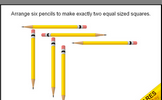#### Two Squares

Using six pencils can you make two equal sized squares?

### 2nd Lesson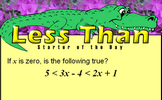#### Less Than

This mathematics lesson starter invites pupils to interpret a three part algebraic inequality.

### 3rd Lesson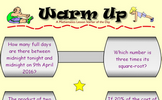#### Warm Up

Four quick Maths questions to warm up the brain.

### 4th Lesson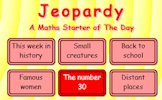#### Jeopardy

Loosely based on the famous television show, how many questions cn you come up with for a given answer?

### 5th Lesson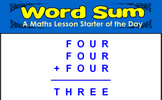#### Word Sum

Each letter stands for a different digit. Can you make sense of word sum?

### 6th Lesson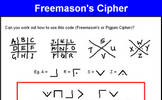#### Freemason's Cipher

Find symmetric words in this ancient cipher.

### 7th Lesson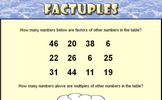#### Factuples

Spot the factors and the multiples amongst the numbers in the grid.

### 8th Lesson#### Inbetween Table

Write down as many multiples of 3.5 as possible in 3.5 minutes.

### 9th Lesson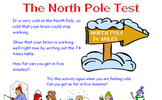#### North Pole Test

This starter requires you to write out a difficult times table.

### 10th Lesson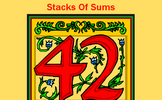#### Stacks of Sums

Write down many different types of calculations which give a particular answer.

### 11th Lesson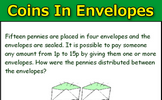#### Coins in Envelopes

Fifteen pennies are placed in four envelopes and the envelopes are sealed. It is possible to pay someone any amount from 1p to 15p by giving them one or more envelopes.

### 12th Lesson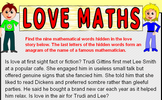#### Love Maths

Find the nine mathematical words hidden in the love story. The last letters of the hidden words form an anagram of the name of a famous mathematician.

Some of the Starters above are to reinforce concepts learnt, others are to introduce new ideas while others are on unrelated topics designed for retrieval practice or and opportunity to develop problem-solving skills.

White Rose ResourcesEnd of block assessments provide a quick progress check at the end of each block of learning to make sure students have understood the content covered. This Scheme of Learning was produced by White Rose Maths and is used here with permission granted on 30th June 2021.For All: